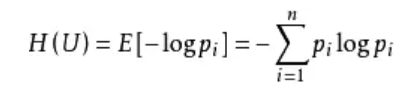# 在OpenCV里实现计算图像信息熵#python 3.7.4,opencv4.1
#蔡军生 https://blog.csdn.net/caimouse/article/details/51749579
#
import numpy as np
import cv2
from matplotlib import pyplot as plt

#计算信息熵
def entropy(labels, base=None):
value,counts = np.unique(labels, return_counts=True)
norm_counts = counts / counts.sum()
print(norm_counts)
base = e if base is None else base
return -(norm_counts * np.log(norm_counts)/np.log(base)).sum()#log(a) b=log (c) b÷log (c) a
#
f = np.array([[119,123,168,119],
[123©️2019 CSDN 皮肤主题: 大白 设计师: CSDN官方博客